# Week 8 Precept

Team 346 pol346.com (Department of Politics, Princeton University)http://princeton.edu/politics
2020-04-08

Data was collected on the corn yield versus rainfall in six U.S. corn-producing states (Iowa, Nebraska, Illinois, Indiana, Missouri, and Ohio), recorded for each year from 1890 to 1927. Although increasing rainfall is associated with higher mean yields for rainfalls up to 12 inches, increasing rainfall at higher levels is associated with no change or perhaps a decrease in mean yield. Why might that be?


# suppress chatty package messages
suppressMessages(library(dplyr))
suppressMessages(library(ggplot2))
suppressMessages(library(stargazer))
suppressMessages(library(janitor))
library(interactions)
library(sjPlot)

knitr::opts_chunk$set(echo = TRUE) 1. Load dataset ex0915  corn <- Sleuth3::ex0915 %>% clean_names() head(corn)  year yield rainfall 1 1890 24.5 9.6 2 1891 33.7 12.9 3 1892 27.9 9.9 4 1893 27.5 8.7 5 1894 21.7 6.8 6 1895 31.9 12.5 1. Plot corn yield vs. rainfall  ggplot(data = corn) + aes(x = rainfall, y = yield) + geom_point() + geom_smooth()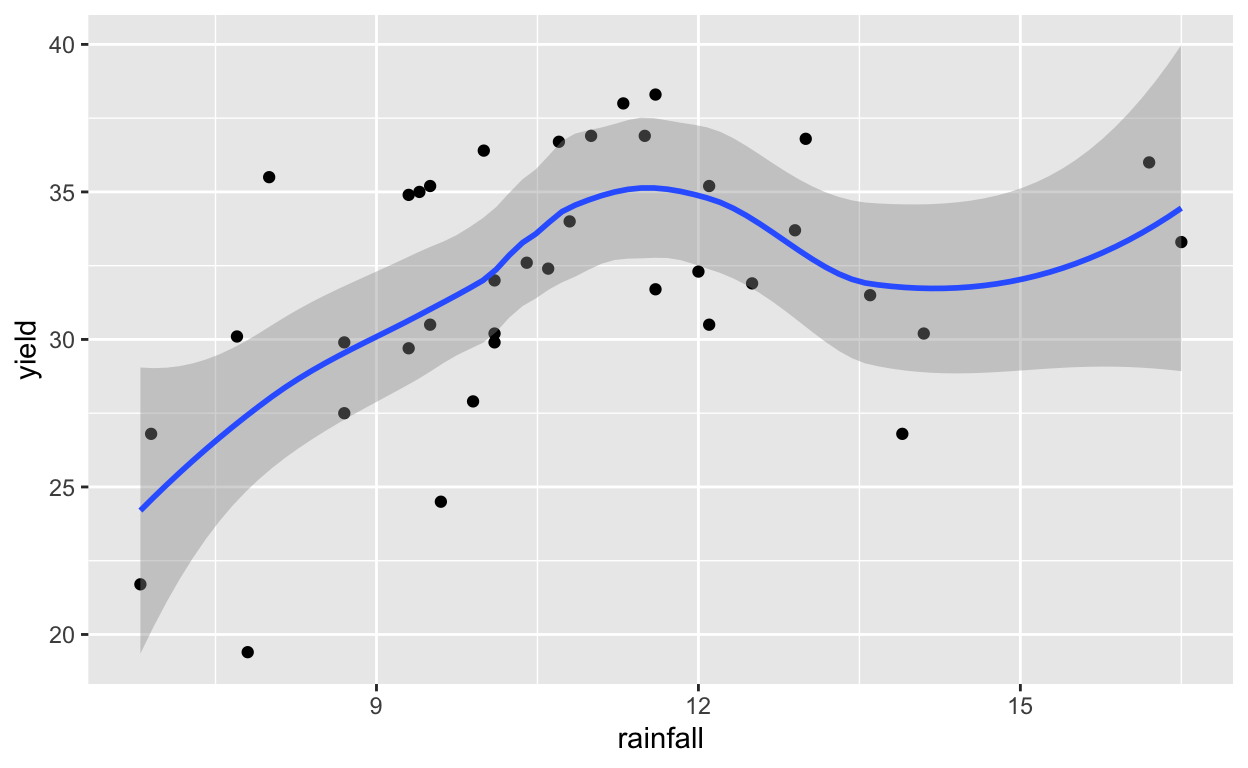1. Does a linear model look appropriate? Why or why not? 2. Fit two regression models, one with yield vs rain, and one with yield vs rain and rain squared.  lm1 <-lm(yield ~ rainfall, data = corn) lm2 <-lm(yield ~ rainfall + I(rainfall^2), data = corn) stargazer( lm1, lm2, type = 'html', digits = 2, header = FALSE )  Dependent variable: yield (1) (2) rainfall 0.78** 6.00*** (0.29) (2.04) I(rainfall2) -0.23** (0.09) Constant 23.55*** -5.01 (3.24) (11.44) Observations 38 38 R2 0.16 0.30 Adjusted R2 0.14 0.26 Residual Std. Error 4.05 (df = 36) 3.76 (df = 35) F Statistic 6.97** (df = 1; 36) 7.38*** (df = 2; 35) Note: p<0.1; p<0.05; p<0.01 1. Use ANOVA to compare the two models. Which does the ANOVA test suggest is preferable?  anova(lm1, lm2)  Analysis of Variance Table Model 1: yield ~ rainfall Model 2: yield ~ rainfall + I(rainfall^2) Res.Df RSS Df Sum of Sq F Pr(>F) 1 36 590.34 2 35 495.53 1 94.807 6.6964 0.01397 * --- Signif. codes: 0 '***' 0.001 '**' 0.01 '*' 0.05 '.' 0.1 ' ' 1 1. Plot residuals vs. year. How do we find residuals? Do you see a pattern? What does it mean?  names(lm2)   "coefficients" "residuals" "effects" "rank"  "fitted.values" "assign" "qr" "df.residual"  "xlevels" "call" "terms" "model"   plot(lm2$residuals ~ corn$year) abline(lm(lm1$residuals ~ corn$year)) abline(h = 0, col = "red")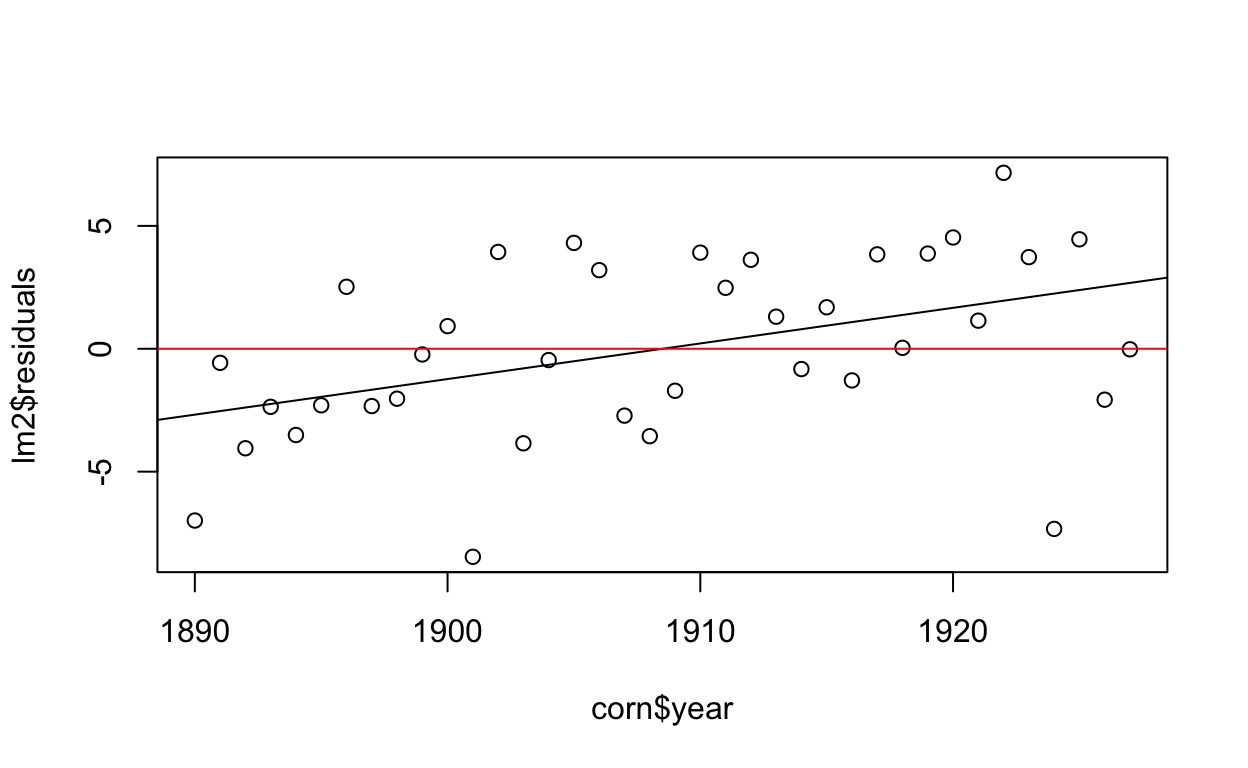1. Are residuals evenly distributed around 0? What do we want in a residual plot? 2. Fit a model of corn yield on rain, rain squared, and year. How have the coefficients changed? How have the standard errors of the coefficients changed? Describe the effect of an increase of one inch of rainfall on the mean yield over the range of rainfall controlling for rainfall-squared and years.  lm3 <-lm(data = corn, yield ~ rainfall + I(rainfall^2) + year) summary(lm3)  Call: lm(formula = yield ~ rainfall + I(rainfall^2) + year, data = corn) Residuals: Min 1Q Median 3Q Max -9.3995 -1.8086 -0.0479 2.4050 5.1839 Coefficients: Estimate Std. Error t value Pr(>|t|) (Intercept) -263.30324 98.24094 -2.680 0.01126 * rainfall 5.67038 1.88824 3.003 0.00499 ** I(rainfall^2) -0.21550 0.08207 -2.626 0.01286 * year 0.13634 0.05156 2.644 0.01229 * --- Signif. codes: 0 '***' 0.001 '**' 0.01 '*' 0.05 '.' 0.1 ' ' 1 Residual standard error: 3.477 on 34 degrees of freedom Multiple R-squared: 0.4167, Adjusted R-squared: 0.3652 F-statistic: 8.095 on 3 and 34 DF, p-value: 0.0003339 1. Now plot residuals vs. year again. Do you still see a pattern? What would explain any difference?  plot(lm3$residuals ~ corn$year) abline(lm(lm3$residuals ~ corn\$year))
abline(h = 0, col = "red")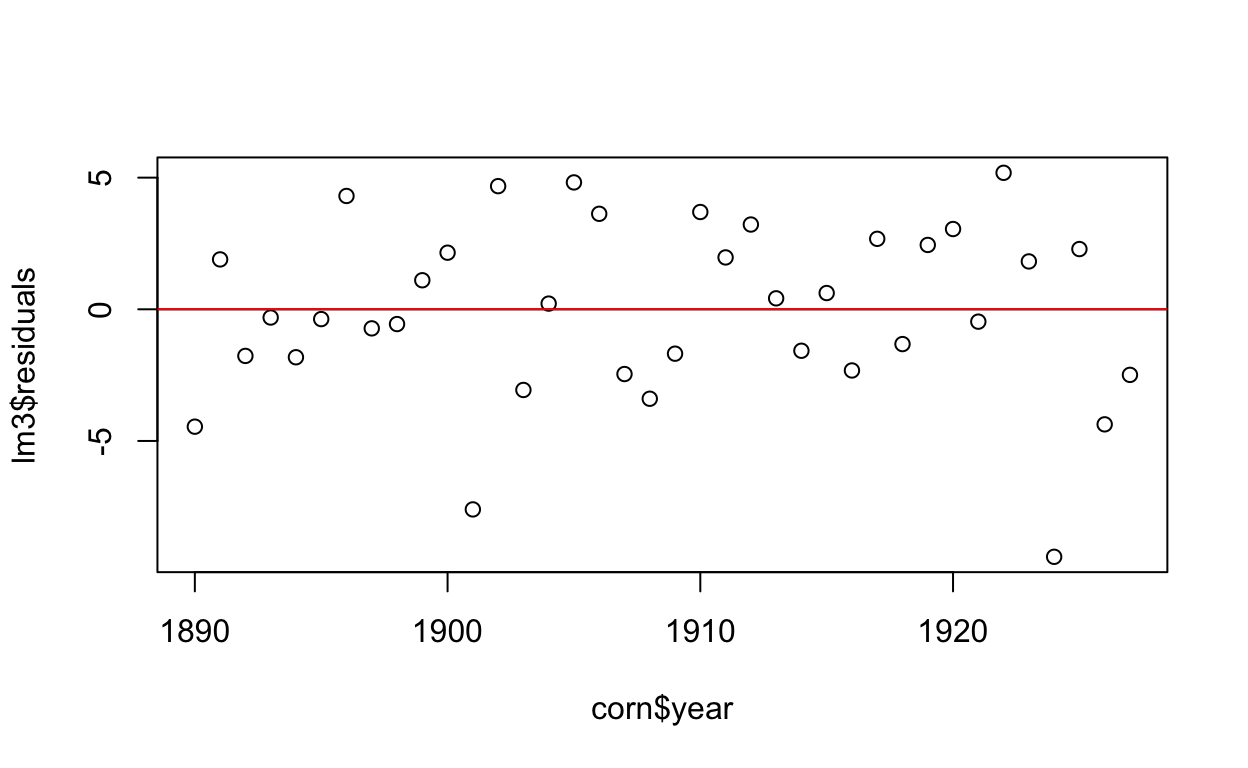1. Fit multiple regression of corn yield on rain, rain squared, and an interaction of year $$\times$$ rainfall. Is the coefficient on the interaction significantly different from zero? How would you interpret this coefficient?

lm4 <-lm(data = corn, yield ~ rainfall + I(rainfall^2)+ rainfall*year)
summary(lm4)

Call:
lm(formula = yield ~ rainfall + I(rainfall^2) + rainfall * year,
data = corn)

Residuals:
Min      1Q  Median      3Q     Max
-6.2969 -2.5471  0.6011  1.9923  5.0204

Coefficients:
Estimate Std. Error t value Pr(>|t|)
(Intercept)   -1.909e+03  4.862e+02  -3.927 0.000414 ***
rainfall       1.588e+02  4.457e+01   3.564 0.001138 **
I(rainfall^2) -1.862e-01  7.198e-02  -2.588 0.014257 *
year           1.001e+00  2.555e-01   3.919 0.000423 ***
rainfall:year -8.064e-02  2.345e-02  -3.439 0.001599 **
---
Signif. codes:  0 '***' 0.001 '**' 0.01 '*' 0.05 '.' 0.1 ' ' 1

Residual standard error: 3.028 on 33 degrees of freedom
Multiple R-squared:  0.5706,    Adjusted R-squared:  0.5185
F-statistic: 10.96 on 4 and 33 DF,  p-value: 9.127e-06
1. Run some pairwise ANOVA comparisons to identify which model you think strikes the right balance between explanatory power and parsimony. HINT: Recall that with ANOVA tests one model must be “nested” within another (e.g., the reduced model should be a simpler version of the full model)

anova(lm3, lm4)

Analysis of Variance Table

Model 1: yield ~ rainfall + I(rainfall^2) + year
Model 2: yield ~ rainfall + I(rainfall^2) + rainfall * year
Res.Df    RSS Df Sum of Sq      F   Pr(>F)
1     34 410.99
2     33 302.55  1    108.44 11.828 0.001599 **
---
Signif. codes:  0 '***' 0.001 '**' 0.01 '*' 0.05 '.' 0.1 ' ' 1
1. Using stargazer(), compare multiple regression results in one table. HINT: to view stargazer output in Console, use type = 'text'. To create narrower columns, use option omit.stat = c("f", "ser") which will drop F-test and Residual Standard Error statistics from output.

stargazer(
lm1, lm2, lm3, lm4,
type      = 'html',
digits    = 2,
omit.stat = c("f", "ser")
)
 Dependent variable: yield (1) (2) (3) (4) rainfall 0.78** 6.00*** 5.67*** 158.84*** (0.29) (2.04) (1.89) (44.57) I(rainfall2) -0.23** -0.22** -0.19** (0.09) (0.08) (0.07) year 0.14** 1.00*** (0.05) (0.26) rainfall:year -0.08*** (0.02) Constant 23.55*** -5.01 -263.30** -1,909.46*** (3.24) (11.44) (98.24) (486.24) Observations 38 38 38 38 R2 0.16 0.30 0.42 0.57 Adjusted R2 0.14 0.26 0.37 0.52 Note: p<0.1; p<0.05; p<0.01
1. Interpreting interaction terms in regression can be hard, plots can help. Run install.packages("interactions") and library(packages).

### interactions::interact_plot versions


interact_plot(
lm3,                 # pick a model to plot
pred = "rainfall",   # this variable will be your x-axis
modx = "year",       # a moderator, i.e. a control we think is important
plot.points = TRUE   # plot the data points
)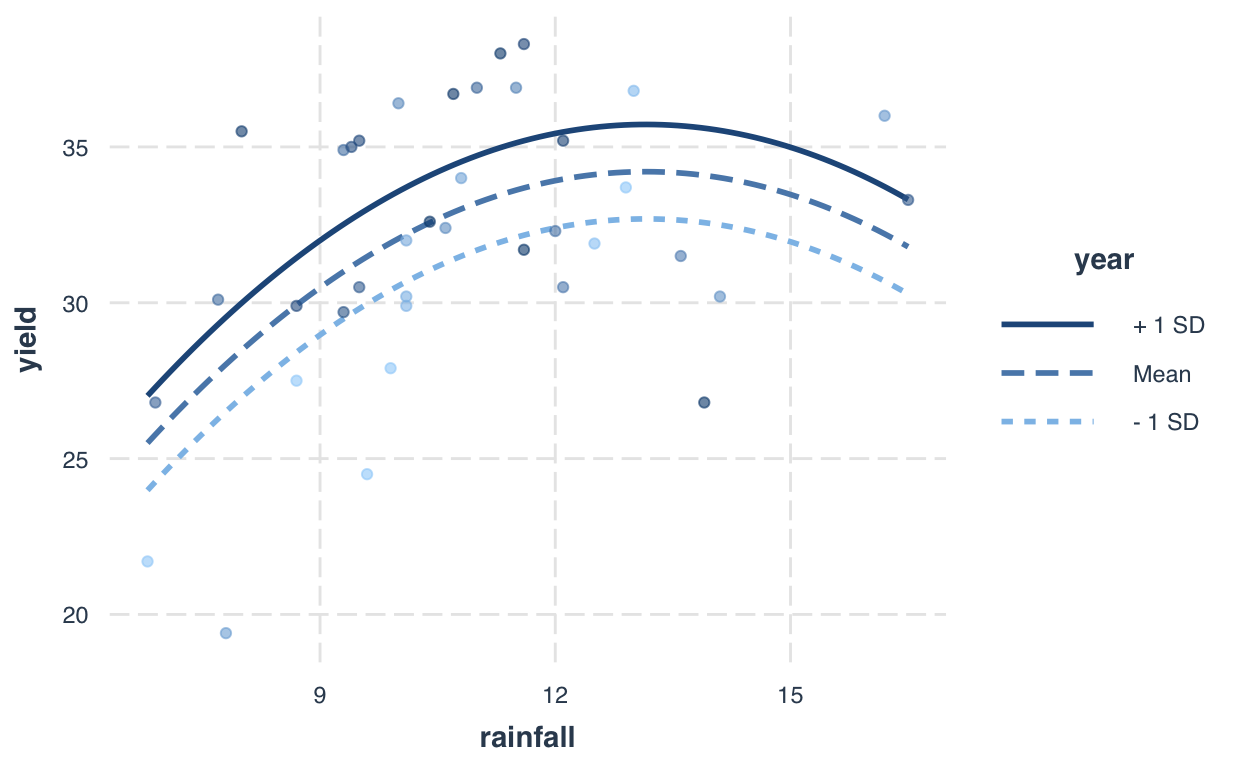interact_plot(
lm4,                 # pick a model to plot
pred = "rainfall",   # this variable will be your x-axis
modx = "year",       # this variable is a control variable
plot.points = TRUE   # plot the data points
)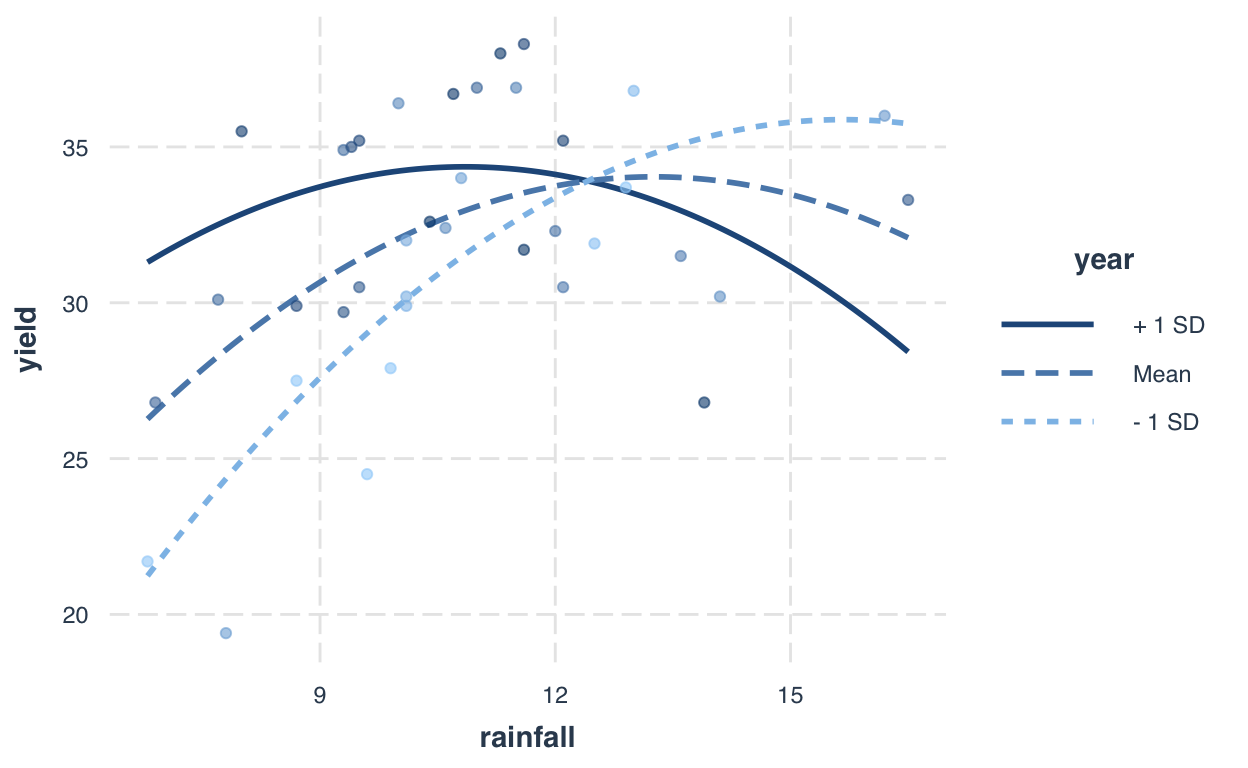### sjPlot::plot_model versions


plot_model(
lm3,                       # pick a model to plot
type      = "pred",        # model type is predicted values of y
terms     = c("rainfall"), # one or more predictors (x)
show.data = TRUE,          # plot the data points
) + theme_minimal()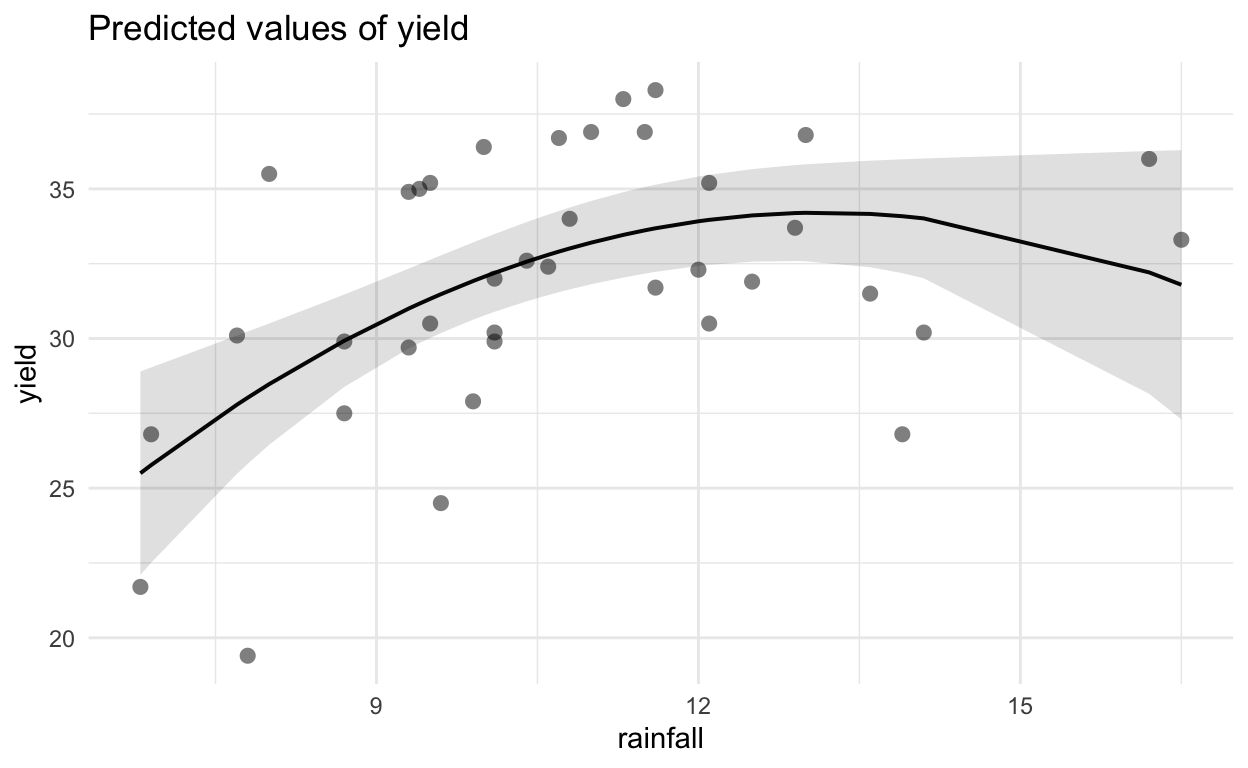plot_model(
lm3,
type      = "pred",
terms     = c("rainfall", "year"),
show.data = TRUE
) + theme_minimal()